###* All email addresses end with "tohoku.ac.jp".

Mathematical Structures I (A01)
(A01-1) Algebraic graph theory, algebraic coding theory, combinatorial design theory
(A01-2) Vertex operator algebras, finite group theory, algebraic combinatorics
Mathematical Structures II (A02)
(A02-1) Geometric function theory, Riemann surfaces, Teichmüller spaces, quasiconformal mappings, complex dynamics
(A02-2) Algebraic combinatorics, spectral graph theory
Mathematical Structures III (A03)
Knot theory, three-manifold theory, quantum topology
Mathematical Structures IV (A04)
Mathematical biology, biomathematics, mathematical modeling
Mathematical System Analysis I (B01)
(B01-1) Combinatorial coding theory, combinatorial design theory
(B01-2) Riemannian geometry, spectral geometry, quantitative geometry
Mathematical System Analysis II (B02)
(B02-1) Quantum probability, infinite dimensional stochastic analysis, complex network
(B02-2) Schrödinger equation, stochastic partial differential equations, numerical analysis
Mathematical System Analysis III (B03)
Theory of partial differential equations, geometric properties of solutions, diffusion equations, elliptic and parabolic equations, the point of view of inverse problems

### Mathematical Structures I (A01)Akihiro Munemasa
Professor
munemasa@math.is.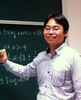Hiroki Shimakura
Associate Professor
shimakura@Yuki Irie
Assistant Professor [RACMaS]
yirie@

Our Laboratory conducts research on mathematical theory with algebraic or discrete approach. The following are our main research subjects.

#### Algebraic combinatoricsThe theory of association schemes has been developed to unify the application of linear programming to coding theory and design theory by Delsarte in 1970's. It generalizes the action of finite groups, and gives a framework for algebraic graph theory, algebraic coding theory and combinatorial design theory. In order to develop algebraic tools for these theories, we investigate applications of algebraic methods to combinatorics, mainly from graph spectra, finite groups, representation theory, linear algebra and optimization.

#### Codes, lattices and vertex operator algebras

A code is a subspace of a finite-dimensional vector space over a finite field. This seemingly simple concept has been widely used to study combinatorial problems using algebraic methods. Codes themselves can also be investigated from tools in number theory, modular forms in particular, via integral lattices. The class of self-dual codes is an interesting class of codes which give rise to unimodular lattices, and are related to the sphere packing problem. Moreover, some vertex operator algebras are constructed from codes and lattices. We investigate the problems of construction and classification of codes, lattices and vertex operator algebras and study their relations.

#### Automorphism groups of vertex operator algebras and the Monster simple group

The Monster simple group is a sporadic simple group, and it is related to number theory and operator algebra theory. We investigate its properties and mysterious phenomena from the view point of automorphism groups of vertex operator algebras. In particular, we focus on algebraic and combinatorial structures related to vertex operator algebras.

### Mathematical Structures II (A02)Toshiyuki Sugawa
Professor
sugawa@math.is.Hajime Tanaka
Associate Professor
htanaka@

The research subject of (A02-1) Sugawa Labo is mainly Complex Analysis.

Even if the data and/or functions are described in terms of real variables, hidden structures may emerge when dealing with them as complex variables. For instance, in the classical problems of moments concerning a sequence of real numbers, the power series formed by the sequence (the generating function) gives us many useful visions to tackle the problems. In such a case, Complex Analysis plays an important role. We are studying analytic functions from the geometric view-point to provide new interpretations to classical results. Moreover, we are interested in quasiconformal mappings, which have recently found many applications in image processing and brain mapping. With the help of computers together with the above knowledge, we are studying modern topics such as Teichmueller spaces, Kleinian groups, Complex Dynamics, and fractals, as well.[The image of the unit disk $|z|<1$ under the conformal mapping $f(z)=z(2-z+z^2)/(2-2z+2z^2)$][A graph of the Riemann zeta function: the height means the absolute value and the color means the argument.]

(A02-2) Tanaka Lab studies various combinatorial objects, mainly by means of algebraic methods.

#### Harmonic analysis on finite groups, association schemes

Homogeneous spaces of finite groups, and Gelfand pairs in particular, have been an active area of research, (partly) because of their connections to, and/or importance in, number theory, orthogonal polynomials and random walks, just to name a few. However, it often turns out to be quite important and natural to shift from "group symmetry" to "combinatorial regularity", and this leads us to the concept of association schemes, which was originally introduced in the design of statistical experiments. We study Gelfand pairs and association schemes through the representations and characters (i.e., spherical functions) of the associated commutative semisimple $\mathbb{C}$-algebras (Hecke algebras and Bose-Mesner algebras), and explore their applications further.

#### Distance-regular graphs, algebraic/spectral graph theory

Distance-regular graphs are finite simple graphs with certain very strong combinatorial regularity. They form a special subclass of association schemes corresponding to two-point homogeneous spaces. Distance-regular graphs are also used as test instances for problems on general graphs, such as random walks and combinatorial optimization. We study distance-regular graphs, in particular their Terwilliger algebras, which are noncommutative semisimple $\mathbb{C}$-algebra introduced by Paul Terwilliger in the early 1990s. Our subjects also include general algebraic/spectral graph theory.

#### Related combinatorics

Various combinatorial objects, including codes and designs, can be viewed as certain subsets of the vertex sets of association schemes and distance-regular graphs. In his monumental thesis in 1973, Philippe Delsarte combined linear programming with the representation theory of the Bose-Mesner algebra to establish a unified theory to study these combinatorial objects, which continues to play a central role in algebraic combinatorics. One of our projects on this topic aims to extend Delsarte's theory based on semidefinite programming and the Terwilliger algebra.[Normalized joint spectral distributions of Cartesian powers of Paley graphs $\mathrm{Paley}(q)$ and their complements]

##### To prospective students

You are welcome to contact me by email if you are interested in the above topics, or more generally, "algebra and combinatorics". The following is a list of (recent) books related to our research:

• A. Terras, Fourier analysis on finite groups and applications, Cambridge University Press, 1999.
• T. Ceccherini-Silberstein, F. Scarabotti, F. Tolli, Harmonic analysis on finite groups, Cambridge University Press, 2008.
• R. A. Bailey, Association schemes: Designed experiments, algebra and combinatorics, Cambridge University Press, 2004.
• A. E. Brouwer, W. H. Haemers, Spectra of graphs, Springer, 2012.
• C. Godsil, K. Meagher, Erdős–Ko–Rado theorems: Algebraic approaches, Cambridge University Press, 2015.

### Mathematical Structures III (A03)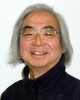Hitoshi Murakami
Professor
hitoshi@

My research interest is in knot theory.

A knot is a knotted circle embedded in the three-dimensional space as shown in the picture Fig. 1 below. You can see intuitively that the two knots on the right are knotted, and three knots are mutually distinct. However, what would you do if you are asked to prove it mathematically?

In knot theory we associate polynomials (possibly with negative powers) to these knots. If these polynomials are different, then we conclude that these knots are different. The picture Fig. 2 shows how to compute the celebrated Jones polynomial. By using the recurrence formula we can compute the Jones polynomials of the three knots Fig. 1, and see that these are indeed different. We often replace intuitive objects such as knots with algebraic objects such as polynomials in knot theory.

By removing a knot itself from the three-space (imagine that we remove a doughnut) and refilling it in another way (twist a doughnut and refill it), we can construct a totally new space. We call such a space a three-manifold. Note that this space is locally the same as the three-space that we live in.

Recently knot theory and three-manifold theory are now developing more and more incorporating ideas such as quantum topology from theoretical physics.[Fig. 1 (from the left) unknot, trefoil, figure-eight knot]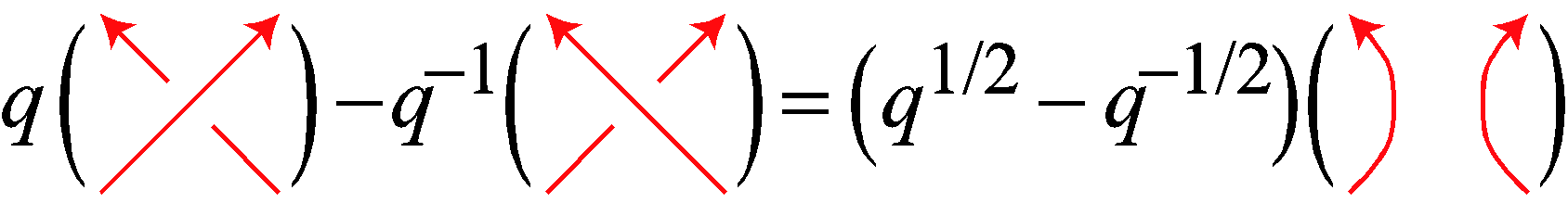[Fig. 2 Jones polynomial]

### Mathematical Structures IV (A04)Hiromi Seno
Professor
seno@math.is.

Seno Labo focuses the theoretical study of mathematical modeling for biological/social phenomena, and the theory on the mathematial structure of modeling.

#### Mathematical Biology

Mathematical biology is an interdisciplinary field growing in a wide range between mathematical and life sciences. Essential theories of mathematics and physics are applied for mathematical analysis in this field. Their development promotes the application of mathematical and theoretical approach for various biological phenomena. Even if the research subject is hard to be attacked with field observation or laboratory experiment, thought experiment could be useful to get some perspectives to conduct field observation or laboratory experiment. Analysis of mathematical model for some problem of theoretical biology can contribute to the systemization of biological theory, and would bring a cue for new biological research. Theory of chaos is one of examples that model analysis in mathematical biology contributed to the development of theories in mathematics and physics.

Principal subject of our study is the mathematical model analysis to make clear or present the point at issue for scientific discussion about real biological/social phenomena, or to promote the advanced theoretical research. Model as simple as possible with only essential factors selected for the targeted phenomenon would provide with scientific (qualitative) discussion about it. Theory of basic modeling could contribute to more advanced modeling for concrete problem about real phenomena. Theoretical research with mathematical model plays an important role for the systemization of biological/social theory.

#### Mathematical Modeling for Biological/Social PhenomenonFocal points in the study of our labo are what theoretical problem about target phenomenon is treated, how the problem is mathematically modeled, what mathematical analysis is applied for the model, how the mathematical result is lead to the discussion in biological/social science. Especially we focus the modeling of mathematical model as simple as possible, which could grasp the nature of phenomenon, and we study the rational consistency/adaptability of mathematical modeling to assumption/hypothesis about biological/social phenomenon.

Generally speaking, mathematical modeling is the process to construct mathematical model with mathematical expression/translation of assumption/hypothesis about the biological/social phenomenon. Estimation of adaptability or translation of such assumption/hypothesis requires knowledge and sense of biological/social science. On the other side, mathematical expression/translation of them requires mathematical knowledge and sense. Therefore, we need an appropriate integration of them for mathematical modeling. This is an interdisciplinary characteristics of the research with mathematical model that has the rational consistency/adaptability to assumption/hypothesis about real phenomenon.

#### Analysis of Mathematical Model

Seno Labo attacks a variety of theoretical problems about biological/social phenomena in wide range of spatial/temporal scale. Some examples of interested research subjects are

• Theory of mathematical modeling for population dynamics in discrete time
• Population dynamics reflected individual behaviour
• Control of ecological system with human intervention
• Effect of spatially fragmented or temporally discontinuous ecological disturbance on population dynamics
• Formation and structure of biological group
• Biological diversity
• Theory of optimal strategy in ecology
• Mathematical modeling for biological phenomena with application of fractal theory

We analyzed basic models which were constructed with application of stochastic process, difference equations, differential equations etc. They were not mainly to be applied for real data analysis, whereas they aimed to make clear the point at scientific issue and to provide some bases of mathematical modeling for advanced/applied researches about real phenomena.

##### To students preparing for an entrance examination

Characteristics of our labo is that we study the mathematical modeling itself as appropriate integration of knowledges and senses in mathematical and biological/social sciences. This can be regarded as an interdisciplinary or applied mathematical viewpoint. Variety of fields or problems contributed by applied mathematics or mathematical biology have been provided from life/social sciences. Researchers with such interdisciplinary mathematical sense shall serve the development in science of coming ages. If you are interested in our labo, please check the web page here to get detail information.

### Mathematical System Analysis I (B01)Masaaki Harada
Professor
mharada@m.Kei Funano
Associate Professor
kfunano@Junya Takahashi
Assistant Professor
t-junya@

(B01-1) Harada Lab studies algebraic coding theory, combinatorial design theory and related combinatorial structures.

#### Algebraic Coding Theory

Our major topic in algebraic coding theory is self-dual codes by an algebraic approach and a combinatorial approach. We are also interested in related combinatorial structures and discrete structures.

#### Combinatorial Design Theory

Combinatorial designs are one of combinatorial structures. Our major topics in combinatorial design theory are symmetric designs, $t$-designs and Hadamard matrices by an algebraic approach and a combinatorial approach.

In (B01-2) Funano Lab. we study Riemannian geometry, spectral geometry, geometry of Banach spaces and metric spaces, and their related topics.

#### Comparison geometry

There are three notions that describe how much our spaces are curved. These are called, sectional curvature, Ricci curvature, and scalar curvature. It is classical that assuming lower bounds of these curvatures corresponds to comparing our space with a simple model space such as euclidean spheres, euclidean spaces, and hyperbolic spaces in some sense. In comparison geometry we study geometric properties by comparing with these spaces. On the other hand there are two notions, called type and cotype in Banach space geometry. These two notions compare Banach spaces with Euclidean spaces in a different fashion. Recently these notions of comparison has been extended to metric spaces and we can now treat wild spaces. Also various methods and notions in convex geometry has been extended to Riemannian manifolds with lower Ricci curvature bounds and we find a close relation between convex geometry and comparison geometry of Ricci curvature. We could expect further development in comparison geometry.

#### Eigenvalues and Eigenfunctions of the Laplacian

The Laplacian is the most basic elliptic second differential operator. It appears not only in the theory of PDE but also in geometry in various situations. It is known that solutions of heat equations, wave equations, and Schrödinger equations are expressed in terms of eigenvalues and eigenfunctions of the Laplacian and so it is important to understand eigenvalues and eigenfunctions to have a better understanding of solutions of these equations. Eigenvalues and eigenfunctions of the Laplacian is closely related with geometric quantities, such as curvature, volumes, distributions of closed geodesics etc. In a discrete setting, it is related with construction of efficient and economical networks and clusterings. In recent years I tried to study eigenvalues and eigenfunctions using optimal transport theory and algebraic topology. I'm also interested in another min-max theory, such as widths, waists etc. These are related with geometric measure theory and theory of minimal surfaces.

##### To prospective students

You are encouraged to send me an email if you have an interest in the above topics and prepare for an entrance examination.

### Mathematical System Analysis II (B02)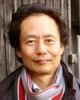Nobuaki Obata
Professor
obata@Reika Fukuizumi
Associate Professor
fukuizumi@math.is.

(B02-1) Obata Labo focuses on various topics in probability theory and infinite dimensional analysis. Moreover, we promote interdisciplinary studies (Applied Mathematics Forum) and international joint research projects.

#### Quantum (Non-commutative) ProbabilityA quantum (non-commutative) extension of the traditional probability theory. Our special interests are spectral analysis of graphs and networks, random matrices, orthogonal polynomials are interesting topics.

#### Complex Networks

Real world complex networks are of great interest in many interdisciplinary research areas. We focus on mathematical foundations as well as applications to life and social science.

#### Stochastic Processes, Stochastic Analysis and Infinite Dimensional Analysis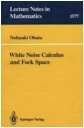Our main concern is to develop the quantum white noise theory with applications to quantum and classical differential equations. Signal analysis, population genetics and sequential data analysis are also within our scope.

(B02-2) Fukuizumi Labo executes advanced researches on PDEs, especially on various nonlinear wave phenomena.

#### Stochastic Partial Differential EquationsWe study, by Ito calculus, the existence, blow-up, asymptotic behavior of solutions of a nonlinear dispersive equation with a stochastic perturbation.

#### Stability of solitary waves and travelling waves

We treat the stability and instability problem of solitary waves. Main tools are variational methods related to nonlinear elliptic equations, and spectral analysis of operators on an infinite dimensional Hilbert space.

#### Numerical Analysis

We are interested in numerical simulations using MATLAB which give effectively some intuitive ideas to solve the phenomena mentioned above rigorously.

### Mathematical System Analysis III (B03)Shigeru Sakaguchi
Professor
sigersak@

Sakaguchi Labo focuses on the study of geometric properties of solutions of partial differential equations. Since solutions are functions, it is natural to want to know their shapes and geometric properties. The main research topics are the following.

#### Stationary isothermic surfaces and stationary critical points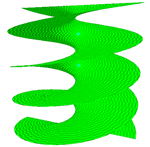To know the shapes of graphs of functions, one may begin by investigating their level surfaces and critical points. In particular, an isothermic surface (a critical point) of the solution of the heat equation is called stationary if its temperature depends only on time (if it is invariant with time). The existence of a stationary isothermic surface (or a stationary critical point) is deeply related to the symmetry of the heat conductor. The right helicoid is an interesting example of stationary isothermic surfaces in the three-dimensional Euclidean space.

#### Interaction between diffusion and geometry of domain

We consider linear and nonlinear diffusion equations (the heat equation, the porous medium equation, etc.). In the problem where the initial value equals zero and the boundary value equals 1, the short-time behavior of solutions is deeply related to the curvatures of the boundary. We also consider parabolic equations which generalize diffusion equations.

#### Shape of solutions of linear and nonlinear elliptic equations

In general, solutions of elliptic equations describe steady states after a sufficiently long time. We consider linear and nonlinear elliptic equations. Liouville-type theorems characterize hyperplanes as graphs of entire solutions with some reasonable restriction. Overdetermined boundary value problems characterize balls, ellipsoids, or some symmetrical domains in general.

#### The point of view of inverse problems

Partial differential equations appear in models describing natural phenomena. It is an interesting problem that characterizes some geometry in some reasonable way from the point of view of inverse problems. The following picture was taken when Sakaguchi stayed in Italy to do research in collaboration with Prof. Magnanini at the university of Florence. Large circular waves were seen on the surface of the circular pond with a fountain.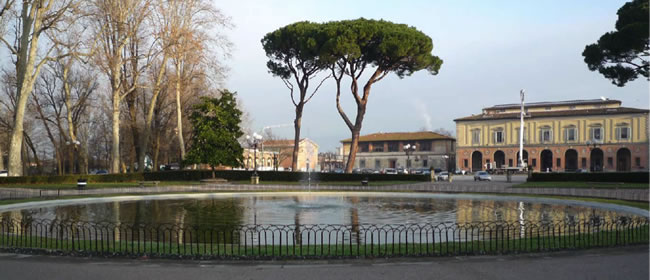The Italian and Japanese mathematical schools have a long history of collaborative research in partial differential equations. The following picture was taken at the Second Italian-Japanese Workshop on Geometric Properties for Parabolic and Elliptic Partial Differential Equations held at Cortona in Italy in June 2011, when Sakaguchi was announcing that the Third Italian-Japanese Workshop would be held in Japan in September 2013.##### To students preparing for an entrance examination

We welcome students who are interested in the qualitative theory of solutions of partial differential equations (geometric properties of solutions etc.) and want to study advanced mathematics more deeply under a basic understanding of mathematics in the undergraduate level (advanced differential and integral calculus, linear algebra, etc.).

Copyright (c) Division of Mathematics. Graduate School of Information Sciences. Tohoku University. All Rights Reserved,